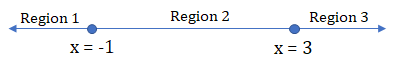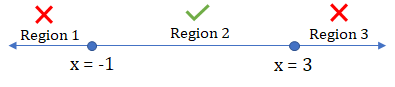GMAT Question of the Day - Daily to your Mailbox; hard ones only

 It is currently 19 Oct 2019, 04:03GMAT Club Daily Prep

Thank you for using the timer - this advanced tool can estimate your performance and suggest more practice questions. We have subscribed you to Daily Prep Questions via email.

Customized
for You

we will pick new questions that match your level based on your Timer History

Track

every week, we’ll send you an estimated GMAT score based on your performance

Practice
Pays

we will pick new questions that match your level based on your Timer History

Not interested in getting valuable practice questions and articles delivered to your email? No problem, unsubscribe here.Solve x^2-2x-3<0

 new topic post reply Question banks Downloads My Bookmarks Reviews Important topics
Author Message
TAGS:

Hide Tags

VPV
Joined: 23 Feb 2015
Posts: 1258

Show Tags00:00

Difficulty:45% (medium)

Question Stats:64% (01:17) correct36% (01:13) wrongbased on 22 sessions

HideShow timer Statistics

Solve $$x^2-2x-3<0$$

1) $$x<-1$$
2) $$x>3$$
3) $$-1<x<3$$

A) 1 only
B) 2 only
C) 1 & 2 only
D) 3 only
E) 1, 2, & 3

_________________
“The heights by great men reached and kept were not attained in sudden flight but, they while their companions slept, they were toiling upwards in the night.”

Do you need official questions for Quant?
3700 Unique Official GMAT Quant Questions
------
SEARCH FOR ALL TAGS
GMAT Club Tests
InternB
Joined: 22 May 2017
Posts: 17
Re: Solve x^2-2x-3<0  [#permalink]

Show Tags

1
The answer here is D.
If you'll factorize the equation then (x-3)(x+1) < 0
This says that one of them is -ve and one is +ve, obviously x+1 > x-3 in all cases so we can say that
1. x+1>0 i.e. +ve => x>-1
2. x-3<0 i.e. -ve => x<3

Thus -1<x<3 is the solution.

Posted from my mobile device
InternB
Joined: 19 Feb 2019
Posts: 20
Re: Solve x^2-2x-3<0  [#permalink]

Show Tags

Factorising the quadratic equation, we get :
x^2 - 2x - 3 => (x+1)(x-3) < 0

Now either (but not both) of these factors can be negative, only then the product will be negative.
Case 1: x+1 < 0 and x-3 > 0 => x<-1 and x>3 which cannot simultaneously be satisfied.
Case 2: x+1 > 0 and x-3 <0 => x>-1 and x<3 => Option D.

Posted from my mobile device
VPV
Joined: 23 Feb 2015
Posts: 1258
Re: Solve x^2-2x-3<0  [#permalink]

Show Tags

CharlieharpeR wrote:
Factorising the quadratic equation, we get :
x^2 - 2x - 3 => (x+1)(x-3) < 0

Now either (but not both) of these factors can be negative, only then the product will be negative.
Case 1: x+1 < 0 and x-3 > 0 => x<-1 and x>3 which cannot simultaneously be satisfied.
Case 2: x+1 > 0 and x-3 <0 => x>-1 and x<3 => Option D.

Posted from my mobile device

hi CharlieharpeR,
Could you explain a little bit the highlighted part?
Thanks__
_________________
“The heights by great men reached and kept were not attained in sudden flight but, they while their companions slept, they were toiling upwards in the night.”

Do you need official questions for Quant?
3700 Unique Official GMAT Quant Questions
------
SEARCH FOR ALL TAGS
GMAT Club Tests
InternB
Joined: 19 Feb 2019
Posts: 20
Re: Solve x^2-2x-3<0  [#permalink]

Show Tags

1
Ok so like I said, for case1, x<-1 and x>3, both these conditions must be true at the same time. Now visualise a number line. All values of x satisfying x<-1 will lie to the left of -1 whereas all values of x satisfying x>3 will lie to the right of 3. Clearly these two intervals do not overlap. So there is no value of x that lies in both these areas and satisfies both conditions simultaneously.

Posted from my mobile device
e-GMAT RepresentativeV
Joined: 04 Jan 2015
Posts: 3074
Re: Solve x^2-2x-3<0  [#permalink]

Show Tags

Solution

Given:
• An inequality of the form $$x^2 – 2x – 3 < 0$$

To find:
• The option choice, that explains the possible value of x

Approach and Working:
We can start solving the inequality by factorizing the expression in the following way:
• $$x^2 – 2x – 3 < 0$$
Or, $$x^2 – 3x + x – 3 < 0$$
Or, $$x (x – 3) + 1 (x – 3) < 0$$
Or, $$(x – 3) (x + 1) < 0$$

Now, at x = -1 and x = 3, the equation becomes 0, and if we plot these two points on the number line, we get total 3 regions to consider:We can check whether the inequality holds true in any specific region, by replacing x with any value belonging to that specific region.
Region 1:
• If x = -2, (x – 3) = negative and (x + 1) = negative
Hence, (x – 3) (x + 1) = negative x negative = positive
But, original expression wise this should be negative.
Hence, Region 1 does not have feasible solutions of x.

Region 2:
• If x = -0, (x – 3) = negative and (x + 1) = positive
Hence, (x – 3) (x + 1) = negative x positive = negative
Also, original expression wise this should be negative.
Hence, Region 2 does have feasible solutions of x.

Region 3:
• If x = 4, (x – 3) = positive and (x + 1) = positive
Hence, (x – 3) (x + 1) = positive x positive = positive
But, original expression wise this should be negative.
Hence, Region 3 does not have feasible solutions of x.Therefore, we can say that feasible values of x exist in the range: -1 < x < 3
Hence, the correct answer is option D.

_________________Re: Solve x^2-2x-3<0   [#permalink] 20 Feb 2019, 20:13
Display posts from previous: Sort by

Solve x^2-2x-3<0

 new topic post reply Question banks Downloads My Bookmarks Reviews Important topics

 Powered by phpBB © phpBB Group | Emoji artwork provided by EmojiOne